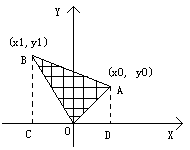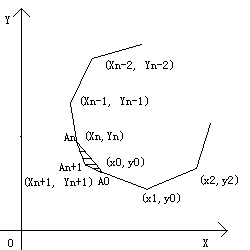# 问题描述

## Problem Description

``````“ 改革春风吹满地,

``````

## Sample Input

``````3 0 0 1 0 0 1
4 1 0 0 1 -1 0 0 -1
0
``````

## Sample Output

``````0.5
2.0
``````

# 问题分析

## Algorithm Analyse

``````S = 0.5 * ( (x0*y1-x1*y0) + (x1*y2-x2*y1) + ... + (xn*y0-x0*yn) )
`````````SABCD = (y0 + y1) × (x0 - x1) ÷ 2
S△OAD = x0 × y0 ÷ 2
S△OBC = (-x1) × y1 ÷ 2

S△OAB = (x0 × y0 + x0 × y1 - x1 × y0 - x1 × y1 - x0 × y0 + x1 × y1) ÷ 2
= (x0 × y1 - x1 × y0) ÷ 2```

``````S = 0.5 × ( (x0×y1-x1×y0) + (x1×y2-x2×y1) + ... + (xn×y0-x0×yn) )
`````````S = 0.5 × ( (x0×y1-x1×y0) + (x1×y2-x2×y1) + ... + (xn×y0-x0×yn) )
S△A0AnAn+1 = 0.5 × ( (X0×Yn-Xn×Y0) + (Xn×Yn+1-Xn+1×Yn) + (Xn+1×Y0-X0×Yn+1) )

∴ S' = S = 0.5 × ( (X0×Y1-X1×Y0) + (X1×Y2-X2×Y1) + ... + (Xn+1×Y0-X0×Yn+1) )
```

``````S = 0.5 × ( (x0×y1-x1×y0) + (x1×y2-x2×y1) + ... + (xn×y0-x0×yn) )。
``````

# 参考源码

redraiment使用Emacs Lisp批量迁移《HDU 2030-2039》